# GMAT Math : DSQ: Calculating range

## Example Questions

2 Next →

### Example Question #11 : Descriptive Statistics

A real estate tycoon wants to test the hypothesis that the percentage of people who own their own home in Florida is the same as the home ownership percentage in Georgia. He states his null hypothesis as 'the Florida percentage is the same as the Georgia percentage' and the alternative hypothesis is 'the percentages are significantly different'. He decides on a 2-tailed test and he samples 400 Florida homes and 600 Georgia homes. His sample results:

Florida - 280 homeowners out of 400 (.7)

Georgia - 384 homeowners out of 600 (.64)

a percentage difference of .06

What is the lowest confidence percentage that will support his rejection of the null hypothesis? Stated another way – what is the highest percentage that will support his acceptance of the null hypothesis?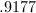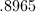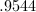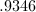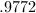Explanation: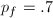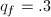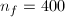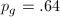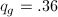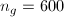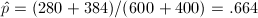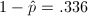standard deviation =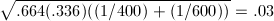0.06 is 2.0 standard deviations from the assumed difference of 0. A z value of 2.0 shows a 1-tailed percentage of .9772 – which is equal to a 2-tailed percentage of .9544

### Example Question #1151 : Data Sufficiency Questions

The average age of our customers is 40 years with a standard deviation of 10 years.

What is the probability that the next customer to enter our store will be between 30 and 50 years old ?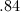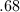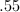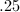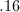Explanation:

The range of 30-50 is plus or minus 1 standard deviation.

The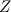value for +1 standard deviation is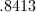thevalue for -1 standard deviation is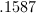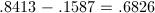### Example Question #13 : Descriptive Statistics

The Z Table is the cornerstone of intro stats. It translates the 'number of standard deviations from the mean' into a percentile (.90, .95. .99. etc.). The table is laid out with the assumption that the test being conducted is a 'one-way' test, meaning that the Z value is greater than some percentile (if Z is positive) or less than some percentile (if Z is negative). Another way of saying this is: the Z table only measures the area under the curve for 1 of the 'tails' (either the extreme right or the extreme left). So, if we have a 2 tailed test for say 95% confidence, then we must put 2.5% in our right tail and 2.5% in our left tail. Conversely, a one tailed test for 95% puts 5% in the right tail only.

Arrange the 5 following confidence intervals in ascending (lowest to highest) order of their Z value:

A) 2 tailed test at 95%

B) 1 tailed test at 95%

C) 1 tailed test at 90%

D) 2 tailed test at 99%

E) 1 tailed test at 99%

CBAED

DECBA

DEABC

ABEDC

CBAED

Explanation:

C) 1 tailed test at 90% - 1.28

B) 1 tailed test at 95% - 1.645

A) 2 tailed test at 95% - 1.96

E) 1 tailed test at 99% - 2.33

D) 2 tailed test at 99% - 2.58

### Example Question #14 : Descriptive Statistics

Ben is given a simple stats problem. Of the 1,000 students at Our Town Community College, 600 are female, 400 are male. The problem asks: if we select 20 students at random, what is the probability that our sample of 20 will contain 10 or 11 or 12 or 13 females.

The simple solution is to go to the binomial table and add up the 4 probabilities of 10, 11, 12 and 13 with a population percentage of 0.6: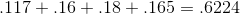However, Ben likes to do things the hard way. He decides to use the normal distribution as an approximation to the binomial. Why? No one knows why. Ben is just Ben.

He gets the Z value and percentile for 9.5 and then obtains the Z value and percentil value for 13.5. He then subtracts the 9.5 percentile from the 13.5 percentile and hopefully ends up with something close to .6224

What is:

the Z value for 9.5

the percentile for 9.5

the Z value for 13.5

the percentile for 13.5

-1.5

.30

.77

.92

-1.34

.23

.71

.85

-1.02

.33

.77

.95

-1.64

.28

.89

.90

-1.14

.13

.68

.75

-1.14

.13

.68

.75

Explanation: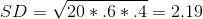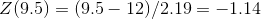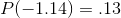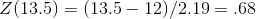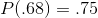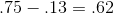### Example Question #15 : Descriptive Statistics

We randomly sampled 175 people in Our Town USA and found that 62.9% of them favored Coke over Pepsi. We decided on a 90% 2 tailed test (z=1.64) and proclaimed that we were 90% confident of the 62.9% plus or minus 6%.

We arrived at the 6% by first calculating the standard deviation: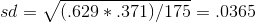and then multiplying .0365 by z (1.64)

What sample size do we need to be plus or minus 4%?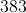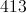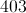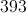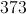Explanation:

sample size =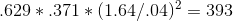### Example Question #16 : Descriptive Statistics

A data set comprises a large number of entries. What is the interquartile range of the set?

Statement 1: The 75th percentile is 745

Statement 2: The median is 556

EITHER statement ALONE is sufficient to answer the question.

Statement 1 ALONE is sufficient to answer the question, but Statement 2 ALONE is NOT sufficient to answer the question.

Statement 2 ALONE is sufficient to answer the question, but Statement 1 ALONE is NOT sufficient to answer the question.

BOTH statements TOGETHER are insufficient to answer the question.

BOTH statements TOGETHER are sufficient to answer the question, but NEITHER statement ALONE is sufficient to answer the question.

BOTH statements TOGETHER are insufficient to answer the question.

Explanation:

Two things are needed to calculate the interquartile range - the first quartile and the third quartile (the median is irrelevant). Statement 1 gives you the latter (the third quartile, by definition, is the 75th percentile) but neither gives you the former.

### Example Question #17 : Descriptive Statistics

A data set comprises 1,000 entries. What is its interquartile range?

Statement 1: The 75th percentile is 64.

Statement 2: The 25th percentile is 30.

EITHER statement ALONE is sufficient to answer the question.

Statement 2 ALONE is sufficient to answer the question, but Statement 1 ALONE is NOT sufficient to answer the question.

BOTH statements TOGETHER are insufficient to answer the question.

BOTH statements TOGETHER are sufficient to answer the question, but NEITHER statement ALONE is sufficient to answer the question.

Statement 1 ALONE is sufficient to answer the question, but Statement 2 ALONE is NOT sufficient to answer the question.

BOTH statements TOGETHER are sufficient to answer the question, but NEITHER statement ALONE is sufficient to answer the question.

Explanation:

The interquartile range is the difference between the third and first quartiles - that is, the 75th percentile and the 25th percentile. Knowing both is necessary and sufficient.

### Example Question #18 : Descriptive Statistics

Seven kids in a kindergarten class have the following ages: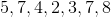.

Amy and Lilly join this kindergarten class. What is the range of the nine kids' ages?

(1) Amy isyears old.

(2) The sum of the ages of both kids is.

Statement (2) ALONE is sufficient, but statement (1) alone is not sufficient

Statement (1) ALONE is sufficient, but statement (2) alone is not sufficient

Statements (1) and (2) TOGETHER are not sufficient

EACH statement ALONE is sufficient

BOTH statements TOGETHER are sufficient, but NEITHER statement ALONE is sufficient

BOTH statements TOGETHER are sufficient, but NEITHER statement ALONE is sufficient

Explanation:

Statement (1) alone does not give us any information to find out the age of Lilly, therefore it is not sufficient.

Statement (2) alone does not give us enough information to determine the ages of each kid. Therefore it is not sufficient.

Statement (1) and (2) give us the following information:

A = 6 and A + L = 13, therefore L = 13 - 6 = 7

With the ages of both kids we can find the new age range = 8 - 2 = 6

### Example Question #19 : Descriptive Statistics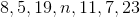What is the value ofin the list above?

(1) The range of the numbers in the list is.

(2)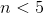Both statements TOGETHER are sufficient, but NEITHER statement ALONE is sufficient.

Both statements TOGETHER are not sufficient.

Statement (2) ALONE is sufficient, but Statement (1) ALONE is not sufficient.

Statement (1) ALONE is sufficient, but Statement (2) ALONE is not sufficient.

Statement (2) ALONE is sufficient, but Statement (1) ALONE is not sufficient.

Statement (1) ALONE is sufficient, but Statement (2) ALONE is not sufficient.

Explanation:

Statement (1) indicates that the range of the numbers is 20. The range is the difference between the highest number and the lowest number. The difference between each pair of numbers in the list is less than 20. Therefore, n is the lowest number in the list. We can then calculate n as follows: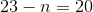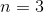Therefore Statement (1) is SUFFICIENT.

Statement (2) states that n<5 which means n is the lowest number in the list. However this information alone is not sufficient to find the value of n.

Therefore Statement (2) is not SUFFICIENT.

Statement (1) ALONE is sufficient, but Statement (2) ALONE is not sufficient.

### Example Question #20 : Descriptive Statistics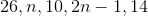What is the range of the numbers in the list above?

(1)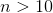.

(2)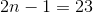.

Statements (1) and (2) TOGETHER are not sufficient.

Statement (2) ALONE is sufficient, but Statement (1) ALONE is not sufficient.

Statement (1) ALONE is sufficient, but Statement (2) ALONE is not sufficient.

Both statements TOGETHER are sufficient, but NEITHER statement ALONE is sufficient.

Each Statement ALONE is sufficient.

Statement (2) ALONE is sufficient, but Statement (1) ALONE is not sufficient.

Explanation:The range of a set of numbers is the difference between the maximum and the minimum values of these numbers. In order to determine the range of these numbers, we need to know the values of n and 2n-1 or at least we should know that n and 2n-1 are not the minimum or the maximum values, meaning n and 2n-1 do not affect the range of these numbers.

(1)Therefore n is not the minimum value in this list. However, we do not if 2n-1 is the maximum value. Statement (1) ALONE is not sufficient.

(2)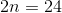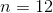n and 2n-1 do not affect the range then. The range of these numbers is: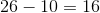Statement (2) ALONE is sufficient.

2 Next →

Tired of practice problems?

Try live online GMAT prep today.